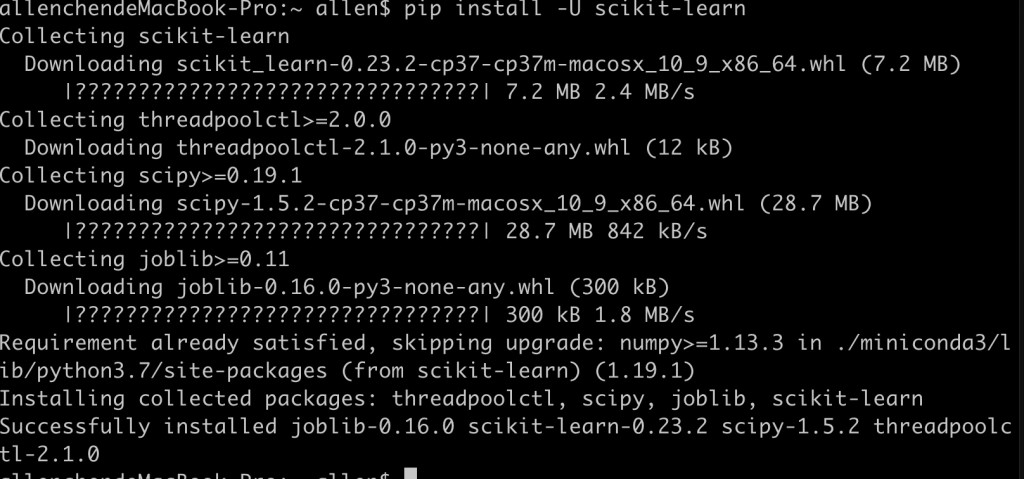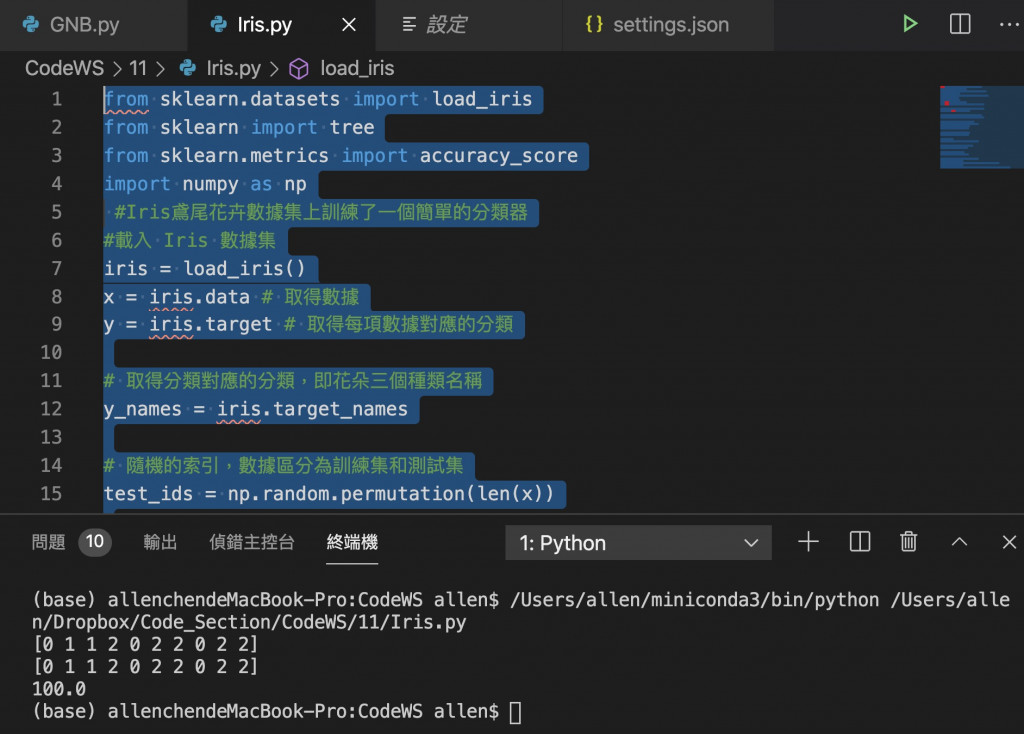DAY 11
0

# 程式實作

## 安裝 scikit-learn

``````pip install -U scikit-learn
``````## Iris鳶尾花卉數據集上訓練了一個簡單的分類器

``````from sklearn.datasets import load_iris
from sklearn import tree
from sklearn.metrics import accuracy_score
import numpy as np
#Iris鳶尾花卉數據集上訓練了一個簡單的分類器
#載入 Iris 數據集
iris = load_iris()
x = iris.data # 取得數據
y = iris.target # 取得每項數據對應的分類

# 取得分類對應的分類，即花朵三個種類名稱
y_names = iris.target_names

# 隨機的索引，數據區分為訓練集和測試集
test_ids = np.random.permutation(len(x))

#把資料和分類區自訓練集和測試集
#最後10個資料係測試集，其他係訓練集
x_train = x[test_ids[:-10]]
x_test = x[test_ids[-10:]]

y_train = y[test_ids[:-10]]
y_test = y[test_ids[-10:]]

# 決策分類器
clf = tree.DecisionTreeClassifier()

# 使用訓練集來訓練我們的分類器
clf.fit(x_train, y_train)

# 測試資料集中進行預測
pred = clf.predict(x_test)

print (pred) # 預測分類，即花朵種類
print (y_test) # 分類
print (accuracy_score(pred, y_test)*100)   # 預測精度
#Fix pylint no member
#https://blog.csdn.net/weixin_44479045/article/details/106324096
``````

# 程式執行成果30天搞懂Python30